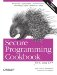# Transforming Elements in a Sequence

Problem

You have a sequence of elements and you have to do something to each one, either in place or as it is copied to another sequence.

Solution

Use the transform or for_each standard algorithms. Both are simple, but allow you to do almost anything you want to the elements in your sequence. See Example 7-9 for an illustration.

Example 7-9. Transforming data

```#include
#include
#include
#include
#include
#include
#include
#include "utils.h" // For printContainer( ): see 7.10

using namespace std;

// Convert a string to upper case
string strToUpper(const string& s) {
string tmp;
for (string::const_iterator p = s.begin( ); p != s.end( ); ++p)
tmp += toupper(*p);
return(tmp);
}

string strAppend(const string& s1, const string& s2) {
return(s1 + s2);
}

int main( ) {

cout << "Enter a series of strings: ";
istream_iterator start(cin);
istream_iterator end;
list lst(start, end), out;

// Use transform with an unary function...
transform(lst.begin( ), lst.end( ),
back_inserter(out),
strToUpper);

printContainer(out);

cin.clear( );

cout << "Enter another series of strings: ";
list lst2(++start, end);
out.clear( );

// ...or a binary function and another input sequence.
transform(lst.begin( ), lst.end( ), lst2.begin( ),
back_inserter(out),
strAppend);

printContainer(out);
}```

Discussion

The obvious function for transforming data is transform. It has two forms. The first form takes a sequence, an output iterator, and an unary functor. It applies the functor to each element in the sequence and assigns the return value to the next element pointed to by the output iterator. The output iterator can be another sequence or the beginning of the originating sequence. In this respect, transform handles both copy-style or in-place transformations.

Here's what the declarations for transform look like:

```Out transform(In first, In last, Out result, UnFunc f);
Out transform(In first1, In last1, In first2, In last2,
Out result, BinFunc f);```

Both versions return an iterator that refers to one past the end of the result sequence.

Using either version is straightforward. To copy strings from one sequence to another, but in uppercase, do as I did in Example 7-9:

```std::transform(lst.begin( ), lst.end( ),
std::back_inserter(out), strToUpper);```

If you want to modify the originating sequence, just pass in the beginning of the sequence as the result iterator:

```std::transform(lst.begin( ), lst.end( ),
lst.begin( ), strToUpper);```

Using two sequences and a binary operation works the same way, and you can use either one of the input sequences as the output sequence.

If you want to transform elements in place, you might want to avoid the overhead of assigning each element to the return value of some function. Or if the functor you want to use modifies its source object, you can use for_each instead:

```void strToUpperInPlace(string& s) {
for (string::iterator p = s.begin( ); p != s.end( ); ++p)
*p = std::toupper(*p);
}
// ...
std::for_each(lst.begin( ), lst.end( ), strToUpperInPlace);```

If what you want to do is change the sequence itself and not necessarily change each of its elements, see Recipe 7.6, where I describe many of the standard algorithms for rearranging the elements in a sequence.

Recipe 7.1 and Recipe 7.6

DiscussionSecure Programming Cookbook for C and C++: Recipes for Cryptography, Authentication, Input Validation & More
ISBN: 0596003943
EAN: 2147483647
Year: 2006
Pages: 241